# Superposition principle — in layman’s terms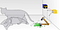Image from Wikipedia article on this topic

While Schrödinger’s cat illustrates superposition, it may trigger some simple questions

The following game will show how we can increase the odds of winning from 50% with a regular fair coin to 100% with a “quantum coin” by

Regular coin game.

Lets first do the game with a regular fair coin.

It is obvious that our chances of winning is 50%. Also, it doesn’t really matter if we opened the box and looked inside at step 4. If we played this game, say a 1000 times, regardless of us looking at the intermediate result at step 4, the chance of winning is always 50%. Figure 1 illustrates this.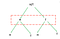Figure 1. Regular coin toss outcomes. The first toss yields heads/tails with 50% chance each. Subsequent tosses yields heads/tails with 50% chance. So chances of winning after second toss is still 50%. It doesn’t matter if we open the box after the first toss — the chances remain unchanged regardless of observing coin after first toss (observation indicated in red)

Quantum coin game.

Lets now play the game with a quantum coin in the box. The quantum coin is similar to the regular coin — meaning it can be in two states, head or tail, and there is a 50% chance of it being in either of these states after an operation equivalent to shaking the box.

We can repeat this game any number of times, and so long we cheat each time and our opponent doesn’t notice, we can win. Lets say, opponent notices that we are always looking at the quantum coin before the first coin toss.

So even though the opponent knows our looking into the quantum box before first shake has something to do with winning, it will not make sense how that can enable us to win, because opening the box after first shake showed the quantum coin behaved exactly like a fair coin.

We can now let in the opponent on our secret. We can confess even we don’t understand it too from a common sense perspective, except the following strategy always works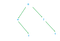Figure 2. Quantum coin toss outcome always yields a tail after second shake when we start off with a head and do not open the box and observe coin after first shake.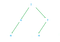Figure 3. Quantum coin toss outcome always yields a head after second shake when we start off with a tail and do not open the box and observe coin after first shake.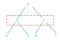Figure 4. Quantum coin toss outcome behaves exactly like a regular coin, regardless of starting off with head or tails and we open the box and observe coin after first shake. The odds of winning is now same as regular coin — 50%.Red box indicates looking at outcome after first shake

So to summarize the strangeness of the quantum coin toss

The mathematical explanation (without equations) for how two of the four branches disappear is quite simple.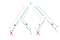Figure 5. Quantum coin — The two paths ultimately leading to heads have opposite and equal values cancelling each other while the two paths ultimately leading to tails add up reinforcing each other. This happens only when the box is not opened after first shake.

This explanation was created based on Scott Aaronson’s blog. There is a book that is largely based on his blog Quantum Computing since Democritus: Scott Aaronson: Amazon.com: Books

For more details on what kind of “shaking operation” is performed on quantum coin etc, notes at the end of the answer to a related question may help How have physicists developed a mathematical model describing quantum phenomenon if we don’t really know what it means?

Originally published at www.quora.com.

Machine learning practitioner

## More from Ajit Rajasekharan

Machine learning practitioner